# AP Statistics : How to do one-sided tests of significance

## Example Questions

### Example Question #1 : How To Do One Sided Tests Of Significance

A pretzel company advertises that their pretzels contain less than 1.0g of of sodium per serving. You take a simple random sample of 10 pretzel servings, and calculate that the mean amount of sodium is 1.20 g, with a standard deviation of 0.1 g.

At the 95% confidence level, does your sample suggest that the pretzels actually have higher than 1.0g of sodium per serving?

No, because t=6.32 and p=0.99993153

Yes, because t= 6.32 and p=0.00013694

No, because t=6.32 and p=0.00006847

Yes, because t= 6.32 and p=0.00006847

No, because t=6.22 and p=0.96593153

Yes, because t= 6.32 and p=0.00006847

Explanation:

This is a one- tailed t-test. It is one-tailed because the question asks whether the pretzel's mean is actually higher, so we are only interested in the right hand tail. We will be using the t-distribution because the population standard deviation is not known.

First we write our hypotheses: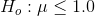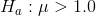Now we need the appropriate formula for a t-test. We will be using standard error because we are working with the standard deviation of a sampling distribution.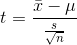Now we fill in the values from our problem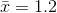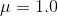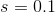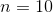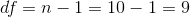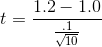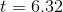Now we must look up the t-critical value, or use technology to find the p-value.

We must find the t-critcal value by finding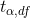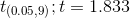Because our test statistic 6.32 is more extreme than our critical value, we reject our null hypothesis and conclude that the pretzels do have a higher mean than 1.0.

If you calculated a p-value using technology, p=0.00006884.

Because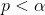, we reject our null hypothesis and conclude that the pretzels do have a higher mean than 1.0 g.

### Example Question #2 : How To Do One Sided Tests Of Significance

James goes to UCLA, and he believes that the atheletes of UCLA are better runners, than the country average. He did a bit of a research and found that the national average time for a two-mile run for college atheletes ismin with a standard deviation ofminute. He then sampledUCLA atheletes and found that their average two-mile time was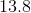minutes.

Is James' data statistically significant? Can we confirm that UCLA atheletes are better than average runners? And if so, to which level of certainty: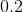,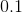,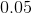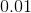Yes, to a certainty ofYes, to a certainty ofYes, to a certainty ofYes, to a certainty ofNo, the data is not statistically significant

Yes, to a certainty ofUsing a Z-test (we have population SD, not sample SD) and a population of, we arrive at a P-value of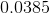, which is lower than, but above.